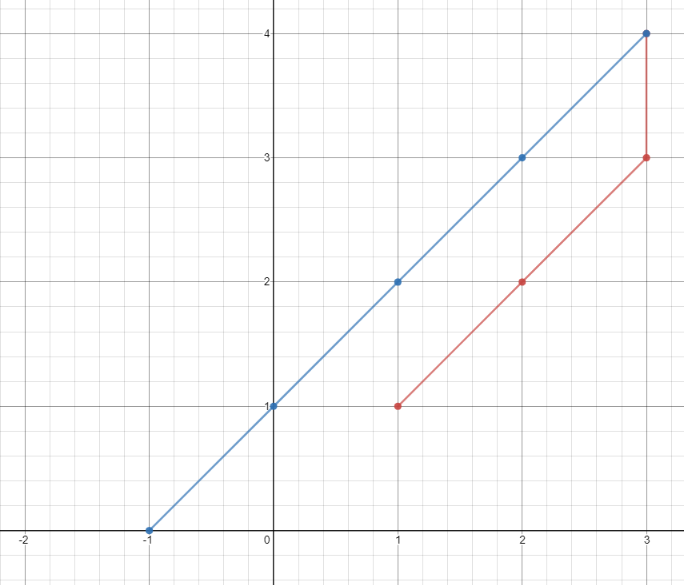1266. Minimum Time Visiting All Points

#### QUESTION:

On a plane there are n points with integer coordinates points[i] = [xi, yi]. Your task is to find the minimum time in seconds to visit all points.

You can move according to the next rules:

In one second always you can either move vertically, horizontally by one unit or diagonally (it means to move one unit vertically and one unit horizontally in one second). You have to visit the points in the same order as they appear in the array.

Example 1:``````Input: points = [[1,1],[3,4],[-1,0]]
Output: 7
Explanation: One optimal path is [1,1] -> [2,2] -> [3,3] -> [3,4] -> [2,3] -> [1,2] -> [0,1] -> [-1,0]
Time from [1,1] to [3,4] = 3 seconds
Time from [3,4] to [-1,0] = 4 seconds
Total time = 7 seconds
``````

Example 2:

``````Input: points = [[3,2],[-2,2]]
Output: 5
``````

Constraints:

``````points.length == n
1 <= n <= 100
points[i].length == 2
-1000 <= points[i], points[i] <= 1000
``````

#### EXPLANATION:

1. 定义一个result来记录结果
2. 获取到i点和i-1的点，两者x和y的最大的delta
3. 累加到result中
4. 返回结果

#### SOLUTION:

``````class Solution {
public int minTimeToVisitAllPoints(int[][] points) {
int result = 0;
for(int i=1;i<points.length;i++)
result+= Math.max(Math.abs(points[i]-points[i-1]),Math.abs(points[i]-points[i-1]));
return result;
}
}
``````
>